# Definition of Standard Deviation

What is Standard Deviation?

Standard deviation measures how widely spread data points are.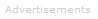If data values are all equal to one another, then the standard deviation is zero.

If a high proportion of data points lie near the mean value, then the standard deviation is small.

An experiment that yields data with a low standard deviation is said have high precision.

If a high proportion of data points lie far from the mean value, then the standard deviation is large.

An experiment that yields data with a high standard deviation is said to have low precision.

Calculating Standard Deviation
The Mean

Standard deviation is the most popular quantitative measure of precision and is measured relative to the mean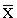.

The mean or average,, is calculated from: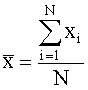Where N is the number of measurements and xi is each individual measurement.is sometimes called the sample mean to distinguish it from the true or population mean, μ.

It's Easier than it Looks: In practice, calculating a mean is much simpler than the somewhat complicated equation above seems to suggest. The equation simply says to add up the values of your measurements and divide by the number of measurements.

Standard Deviation

The standard deviation, s, is a statistical measure of the precision for a series of repeated measurements. The advantage of using s to quote uncertainty in a result is that it has the same units as the experimental data.

Under a normal distribution, (± one standard deviation) encompasses 68% of the measurements and (± two standard deviations) encompasses 96% of the measurements. Standard deviation is calculated from: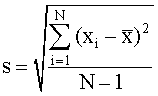Where N is the number of measurements, xi is each individual measurement, andis the mean of all measurements.

The quantity (xi -) is called the "residual" or the "deviation from the mean" for each measurement. The quantity (N - 1) is called the "degrees of freedom" for the measurement.Relative standard Deviation

The relative standard deviation (RSD) is useful for comparing the uncertainty between different measurements of varying absolute magnitude. The RSD is calculated from the standard deviation, s, and is commonly expressed as parts per thousand (ppt) or percentage (%):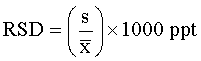The %-RSD is also called the "coefficient of variance" or CV.

Other Measures of Precision

The quantitative measures of precision described above are the most common for reporting analytical results. You might encounter other measures of precisions, and several other quantities are listed here for completeness.

Standard ErrorVariance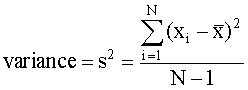The advantage of working with variance is that variances from independent sources of variation may be summed to obtain a total variance for a measurement.

All of the equations above are intended to obtain the precision of a relatively small numbers of repeated measurements. For 20 or more measurements you need to use:

The True or Population Standard Deviation

This is given the symbol sigma: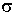The equation is: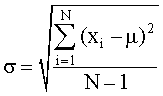Search the Dictionary for More Terms